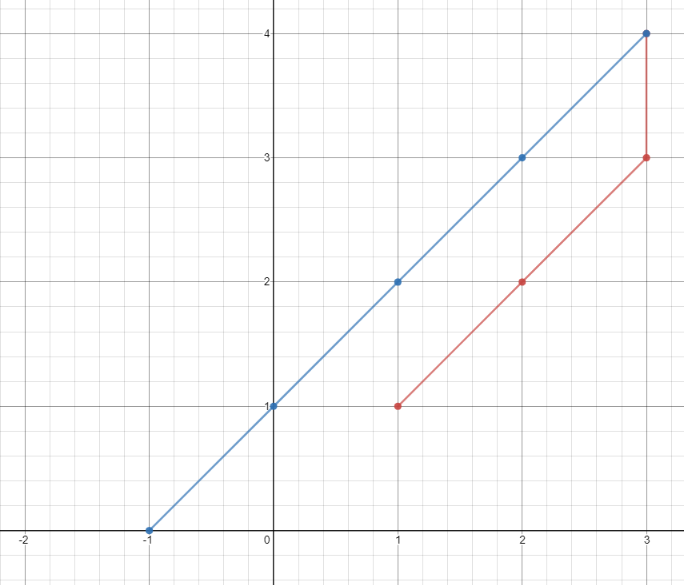# 1266. Minimum Time Visiting All Points

On a plane there are `n` points with integer coordinates `points[i] = [xi, yi]`. Your task is to find the minimum time in seconds to visit all points.

You can move according to the next rules:

• In one second always you can either move vertically, horizontally by one unit or diagonally (it means to move one unit vertically and one unit horizontally in one second).
• You have to visit the points in the same order as they appear in the array.

Example 1:Input:

``` points = [[1,1],[3,4],[-1,0]]
```

Output:

``` 7
```

Explanation:

`One optimal path is`

[1,1]

` -> [2,2] -> [3,3] ->`

[3,4]

`-> [2,3] -> [1,2] -> [0,1] ->`

[-1,0]

```
Time from [1,1] to [3,4] = 3 seconds
Time from [3,4] to [-1,0] = 4 seconds
Total time = 7 seconds```

Example 2:

Input:

``` points = [[3,2],[-2,2]]
```

Output:

``` 5
```

Constraints:

• `points.length == n`
• `1 <= n <= 100`
• `points[i].length == 2`
• `-1000 <= points[i], points[i] <= 1000`

```class Solution {
public int minTimeToVisitAllPoints(int[][] points) {
int result = 0;
int prev_x = points;
int prev_y = points;
for (int i = 1; i < points.length; i ++){
int x = points[i];
int y = points[i];

int diff_x = Math.abs(x - prev_x);
int diff_y = Math.abs(y - prev_y);

result += Math.min(diff_x,diff_y)+Math.abs(diff_x-diff_y);

prev_x = x;
prev_y = y;
}
return result;
}
}```